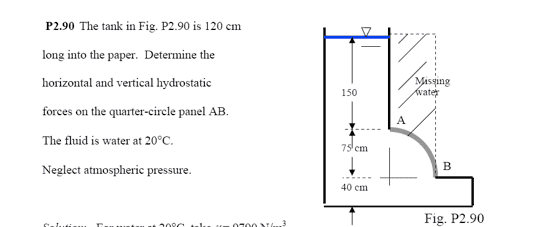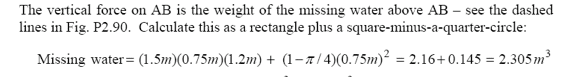# Hydrostatics Problem (Why is this Wrong?)In calculating the vertical force component, you want to find the weight of the missing water.

This should be simple: Find the Volume of the missing water and multiply by specific weight.

Why is my volume calculation incorrect? I am using the volume of the rectangular prism whose top coincides with the surface and whose base coincides with B. Then I subtract the volume of the quarter cylinder:

$$V_{Tot}=V_{rect}-.25V_{cyl}$$

$$V_{rect}=(1.5+0.75)(0.75)(1.2)$$

$$.25*V_{cyl}=.25*(\pi (0.75)^2(1.2)$$

But this does not give the correct volume. The solution says to use:I am failing miserably to see how my method is different from theirs? Am I messing up a dimension here?

Aaahhhhhhhh! My brain!

Edit: How does what they wrote even make any sense?

The first term has dimensions of m^3 and the second has dimensions of m^2 !

Not to mention, if you put those numbers into your calculator they do not equal what they are saying they equal. WTF

Last edited:
It looks like the second term in their solution has a typo: They left off the factor of 1.2m.

It looks like the second term in their solution has a typo: They left off the factor of 1.2m.

Yeah. I think that makes it equivalent to mine if you tack that on.

Sometimes it does not help to be able to check your answers. :grumpy: